Tidytuesday Avatar
Aug 12, 2020

# Tidytuesday Avatar 2020-08-12

``````library(tidytuesdayR)
library(tidyverse)
library(tidymodels)
library(stringr)``````

### Getting data

``````avatar <- readr::read_csv('https://raw.githubusercontent.com/rfordatascience/tidytuesday/master/data/2020/2020-08-11/avatar.csv')

### Data exploration

``````avatar_summary_chapter<-
avatar %>% group_by(book_num,chapter_num) %>%
summarize(mean_rating = mean(imdb_rating, na.rm = TRUE)) %>%
as_tibble() %>%
mutate_if(is.character,as.factor)

avatar_summary_chapter %>% group_by(book_num) %>%
summarize(mean_rating = mean(mean_rating, na.rm = TRUE))  %>%
ggplot(aes(book_num,mean_rating)) +
geom_bar(stat='identity')+
theme(legend.position='none')+
labs(title = "Imdb rating acroos the three books",
y = "Mean imdb rating", x = "Book number")``````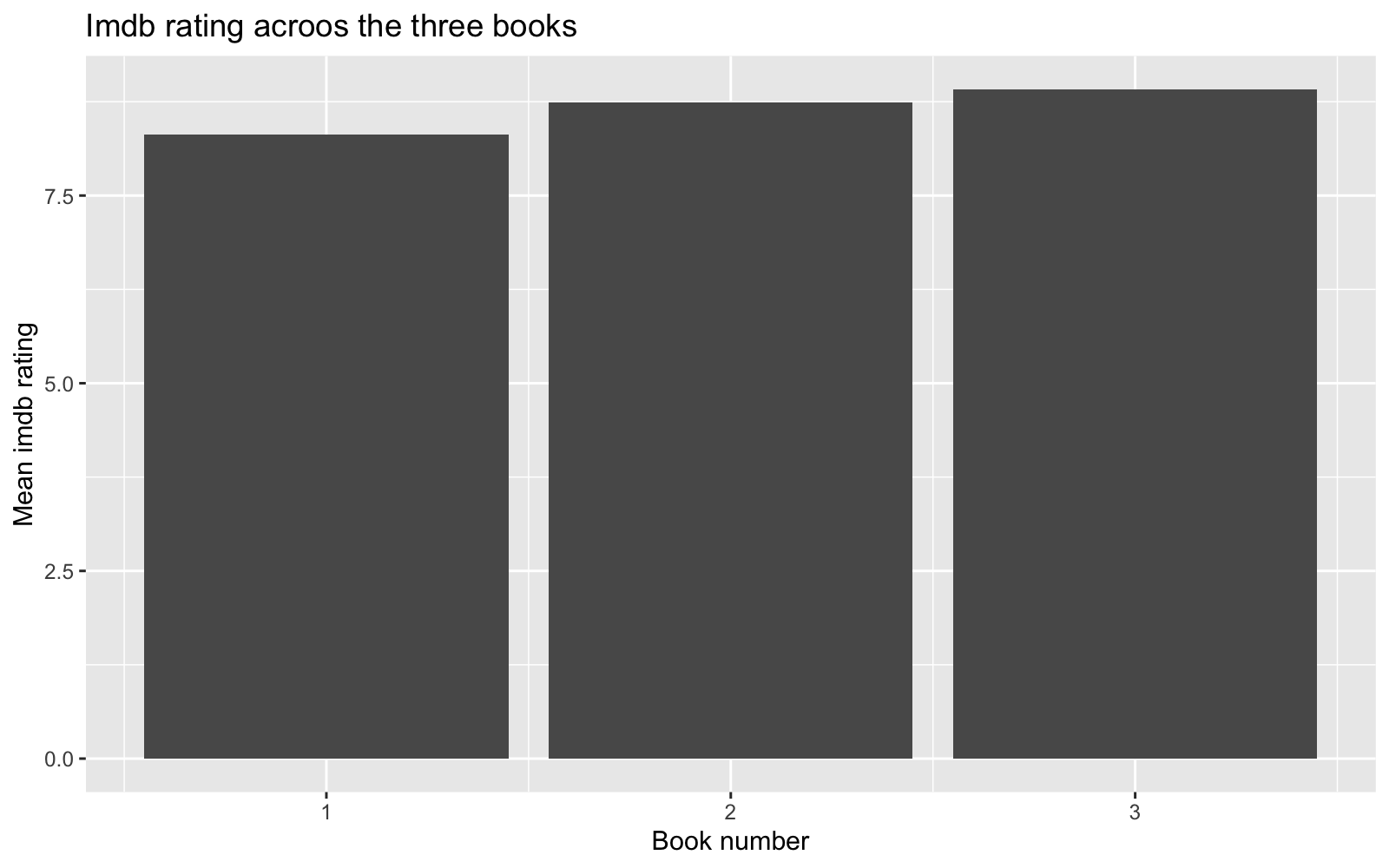``````avatar_summary_character<-avatar %>% group_by(character,book_num) %>%
summarize(mean_rating = mean(imdb_rating)) %>%
as_tibble() %>%
mutate_if(is.character,as.factor)

avatar_summary_character %>%
ggplot(aes(book_num,mean_rating,group=character))+
geom_line(aes(group = character)) +
geom_point(aes(color=character))+
theme(legend.position='none')+
labs(title = "Imdb rating associated to each character",
y = "Mean imdb rating", x = "Book number")``````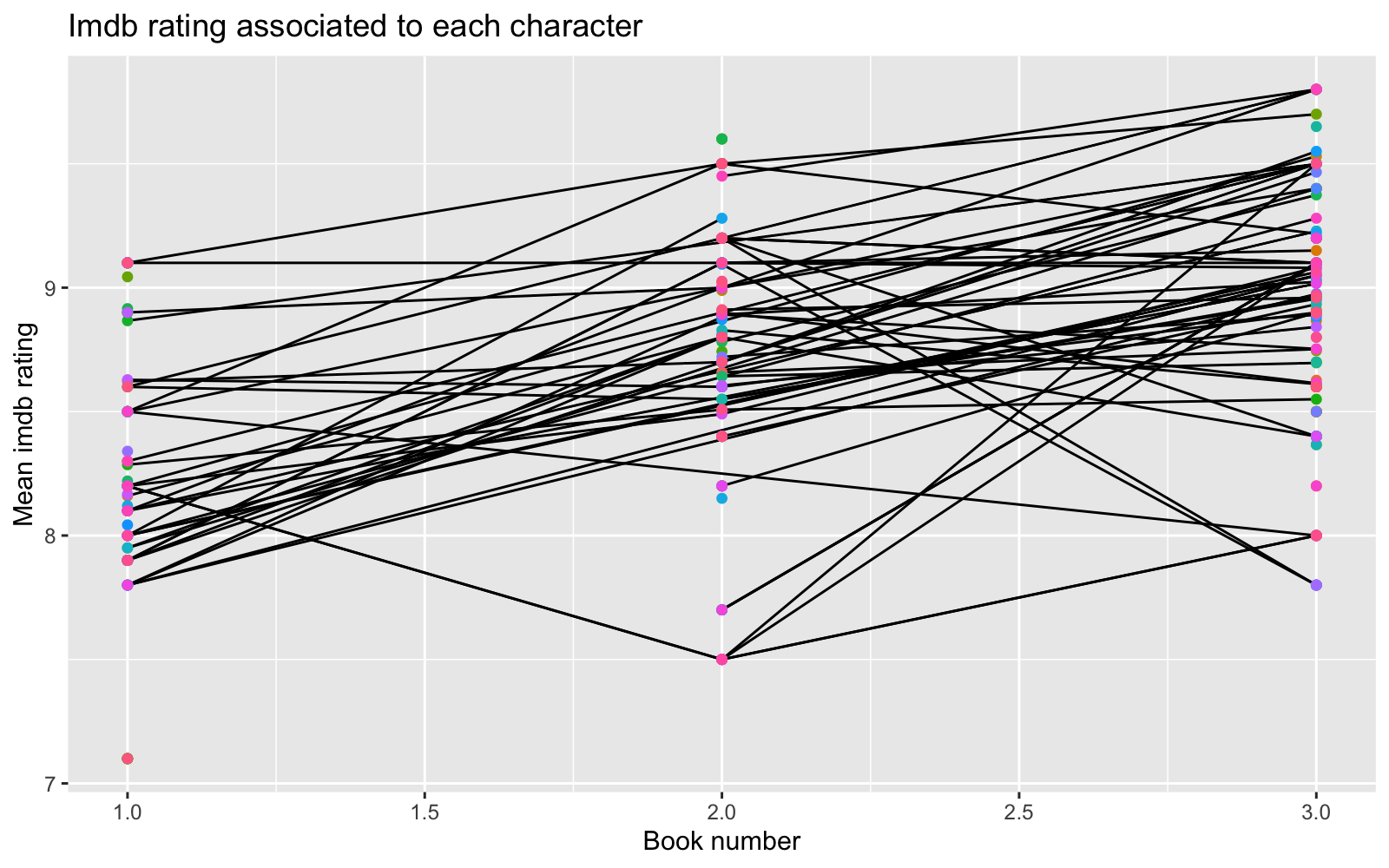``````avatar_summary_writer<-avatar %>% group_by(writer,book_num) %>%
summarize(mean_rating = mean(imdb_rating,na.rm=TRUE)) %>%
as_tibble()
# mutate_if(is.character,as.factor)

avatar_summary_writer\$writer <-avatar_summary_writer\$writer %>%  str_trunc(.,30,"right")
avatar_summary_writer<-avatar_summary_writer %>% mutate_if(is.character,as.factor)

avatar_summary_writer %>%
ggplot(aes(book_num,mean_rating))+
geom_line(aes(group = writer, color=writer)) +
geom_point(aes( color=writer))+
theme(legend.position='top')+
labs(title = "Imdb rating associated to each writer",
y = "Mean imdb rating", x = "Book number")``````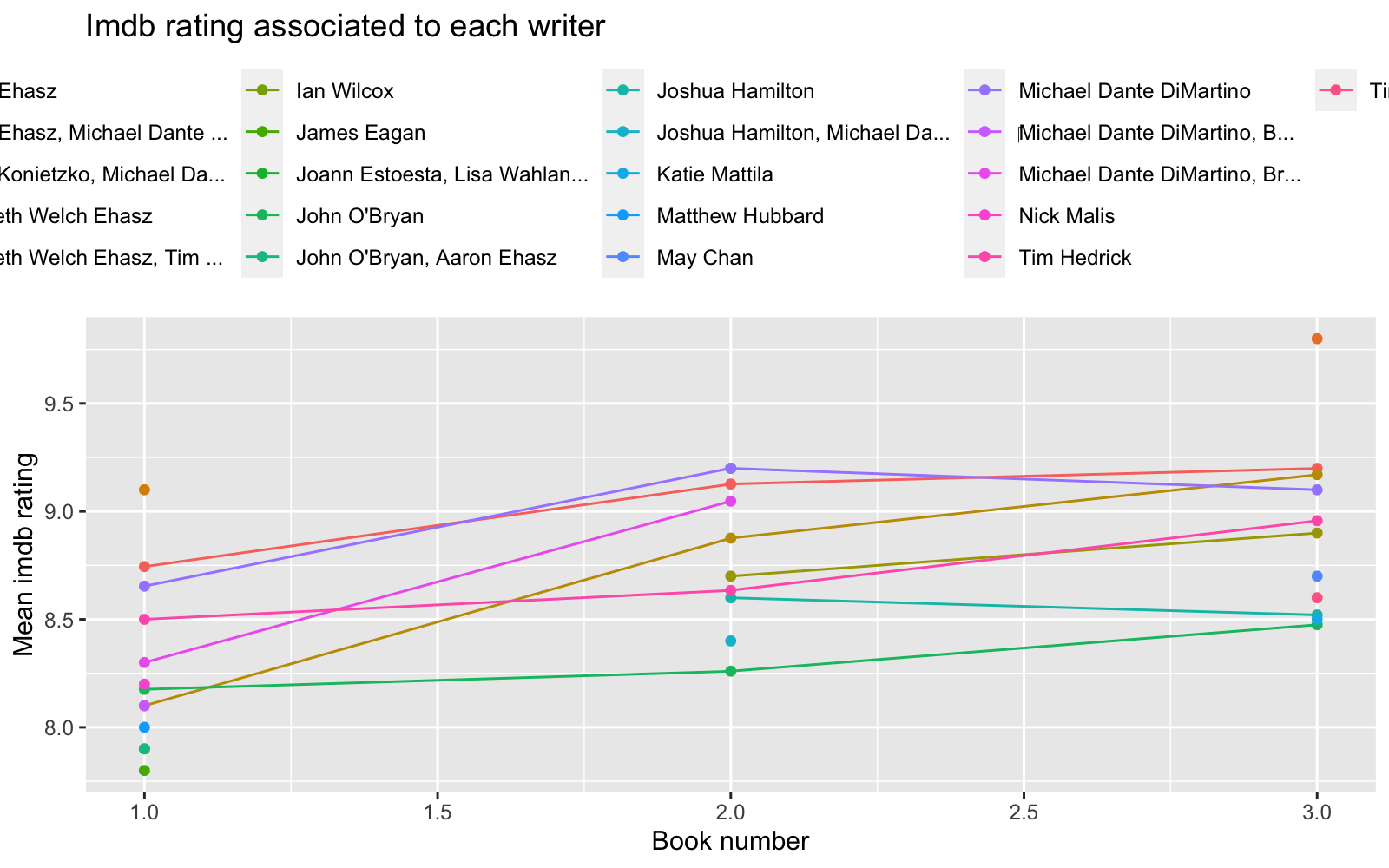``````avatar_summary_director<-avatar %>% group_by(director,book_num) %>%
summarize(mean_rating = mean(imdb_rating,na.rm=TRUE)) %>%
as_tibble() %>%
mutate_if(is.character,as.factor)

avatar_summary_director %>%
ggplot(aes(book_num,mean_rating))+
geom_line(aes(group = director, color=director)) +
geom_point(aes( color=director))+
theme(legend.position='top')+
labs(title = "Imdb rating associated to each director",
y = "Mean imdb rating", x = "Book number")``````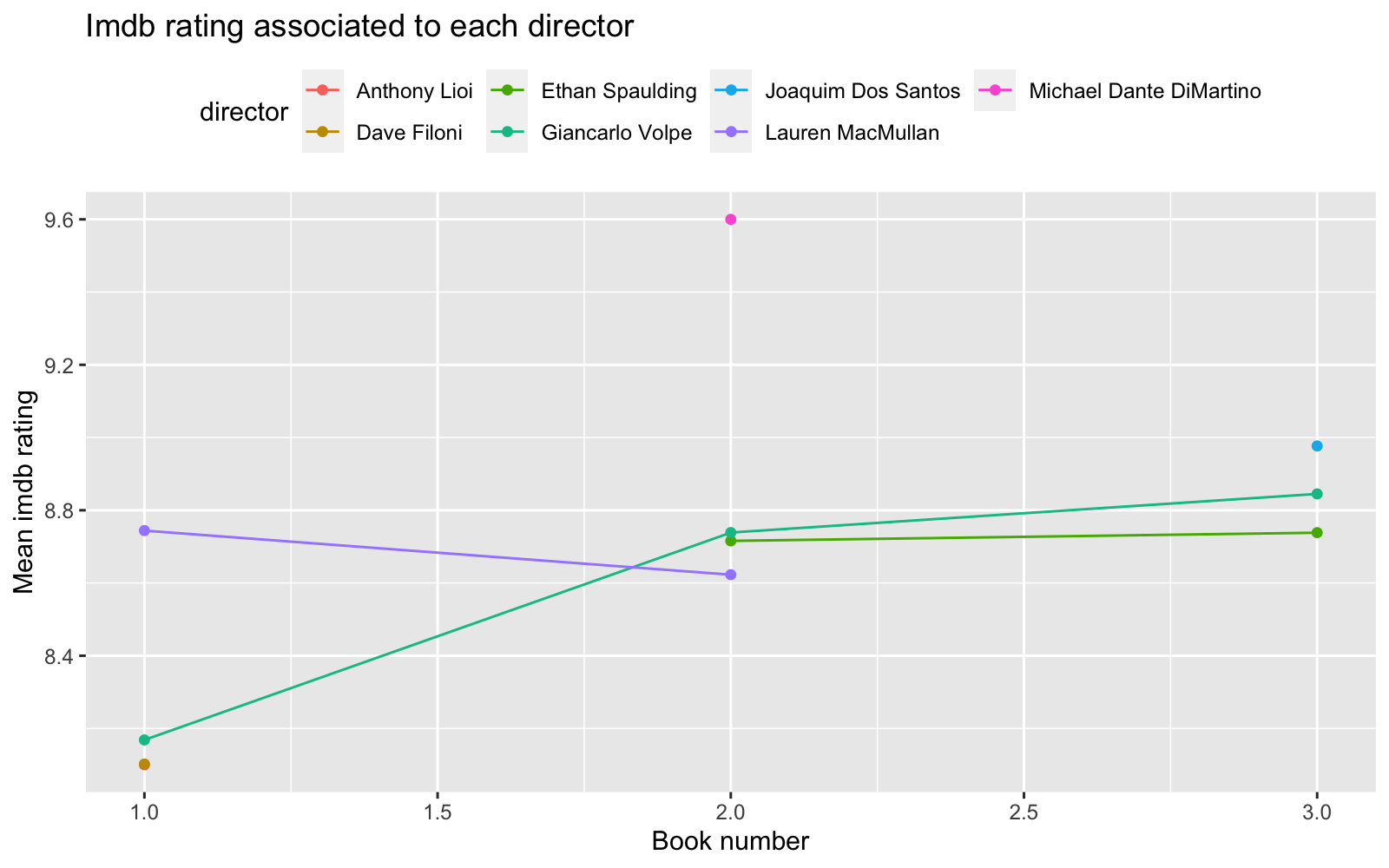``````avatar_summary_director_writer<-avatar %>% group_by(writer,director,book_num) %>%
summarize(mean_rating = mean(imdb_rating,na.rm=TRUE)) %>%
as_tibble() %>%
mutate(combination=paste(writer,director,sep="."))

avatar_summary_director_writer\$combination <-avatar_summary_director_writer\$combination %>%  str_trunc(.,30,"right")
avatar_summary_director_writer<-avatar_summary_director_writer %>% mutate_if(is.character,as.factor)

#mutate_if(is.character,as.factor)

avatar_summary_director_writer %>%
drop_na() %>%
ggplot(aes(mean_rating,combination))+
geom_bar(position="dodge", stat="identity")+
theme(legend.position='top')+
labs(title = "Imdb rating associated to writer-director combos",
x = "Mean imdb rating", y = "")``````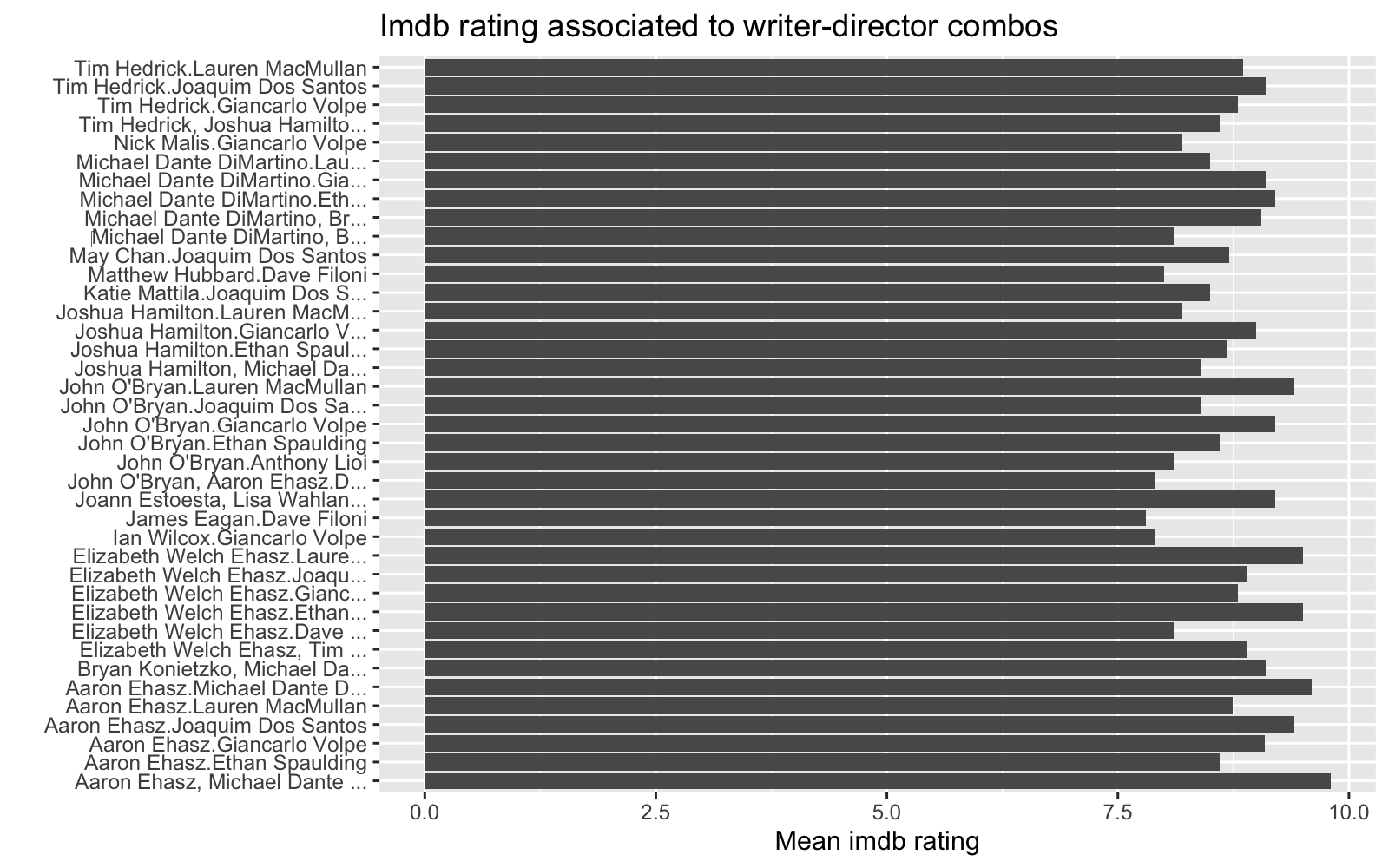It looks like episodes associated to later books got a slightly better Imdb rating. Some characters tend to be associated with better Imdb rating. Also it seems that episodes based on chapters written by some authors get very different Imdb rating. For instance episodes based on Book 1 chapters written by some authors got low Imdb rating and those authors did not participate writing chapters in Book 2 and Book 3. It looks like some star author(s) were hired for the last book, and those chapters got highest Imdb rating. Finally, some directors that directed episodes based on Book 2 and 3 got pretty high rating, and it looks like those directors were hired for one season only.

Some writers may be writing more about popular characters and likewise some episodes directed by some directors may have higher presence of some popular characters, which may be pushing higher Imdb rates these artist may eventually receive. Maybe a combination of best writers and directors gives the top rates.

Next question we have is what parameters are driving Imdb rating?

``````avatar_simple<-avatar %>%
select(character,book_num,chapter_num,writer,director,imdb_rating) %>%
dplyr::mutate(book_num = replace_na(book_num, 0),
chapter_num = replace_na(chapter_num, 0),
imdb_rating= replace_na(imdb_rating, 0),
character= replace_na(character, 0),
) %>%
dplyr::filter(imdb_rating!=0) %>%
mutate(imdb_rating = case_when(
imdb_rating > 8.75 ~ "high",
imdb_rating < 8.75 ~ "low")) %>% unique()%>%
na.omit() %>%
mutate_if(is.character, factor) %>%

janitor::clean_names() %>%
mutate(character = fct_lump(character,100))

avatar_simple %>% count(imdb_rating)``````
``````## # A tibble: 2 x 2
##   imdb_rating     n
##   <fct>       <int>
## 1 high          474
## 2 low           475``````

We can consider an Imdb rating as low if this is <8.75 and high if Imdb rating is >8.75

### Building models with recipes

``````avatar_split <- initial_split(avatar_simple, strata = imdb_rating)
avatar_train <- training(avatar_split)
avatar_test <- testing(avatar_split)

avatar_rec <- recipe(imdb_rating ~ ., data = avatar_train) %>%
# update_role(id, new_role = "ID") %>%
step_dummy(all_nominal(), -all_outcomes()) %>%
step_zv(all_numeric()) %>%
step_normalize(all_numeric())

avatar_prep <- avatar_rec %>%prep()
avatar_juiced <- juice(avatar_prep)

glm_spec <- logistic_reg() %>%
set_engine("glm")

glm_fit <- glm_spec %>%
fit(imdb_rating ~ ., data = avatar_juiced)

knn_spec <- nearest_neighbor() %>%
set_engine("kknn") %>%
set_mode("classification")

knn_fit <- knn_spec %>%
fit(imdb_rating ~ ., data = avatar_juiced)

tree_spec <- decision_tree() %>%
set_engine("rpart") %>%
set_mode("classification")

tree_fit <- tree_spec %>%
fit(imdb_rating ~ ., data = avatar_juiced)``````

### We can now evaluate the models with resampling

``````set.seed(2020)
folds <- vfold_cv(avatar_train, strata = imdb_rating)``````

We can estimate the models metrics

``````set.seed(234)
glm_rs <- glm_spec %>%
fit_resamples(
avatar_rec,
folds,
metrics = metric_set(roc_auc, sens, spec),
control = control_resamples(save_pred = TRUE)
)

set.seed(234)
knn_rs <- knn_spec %>%
fit_resamples(
avatar_rec,
folds,
metrics = metric_set(roc_auc, sens, spec),
control = control_resamples(save_pred = TRUE)
)

set.seed(234)
tree_rs <- tree_spec %>%
fit_resamples(
avatar_rec,
folds,
metrics = metric_set(roc_auc, sens, spec),
control = control_resamples(save_pred = TRUE)
)

glm_rs %>%
unnest(.predictions) %>%
mutate(model = "glm") %>%
bind_rows(knn_rs %>%
unnest(.predictions) %>%
mutate(model = "knn")) %>%
group_by(model) %>%
roc_curve(imdb_rating, .pred_high) %>%
ggplot(aes(x = 1 - specificity, y = sensitivity, color = model)) +
geom_line(size = 1.5) +
geom_abline(
lty = 2, alpha = 0.5,
color = "gray50",
size = 1.2
)``````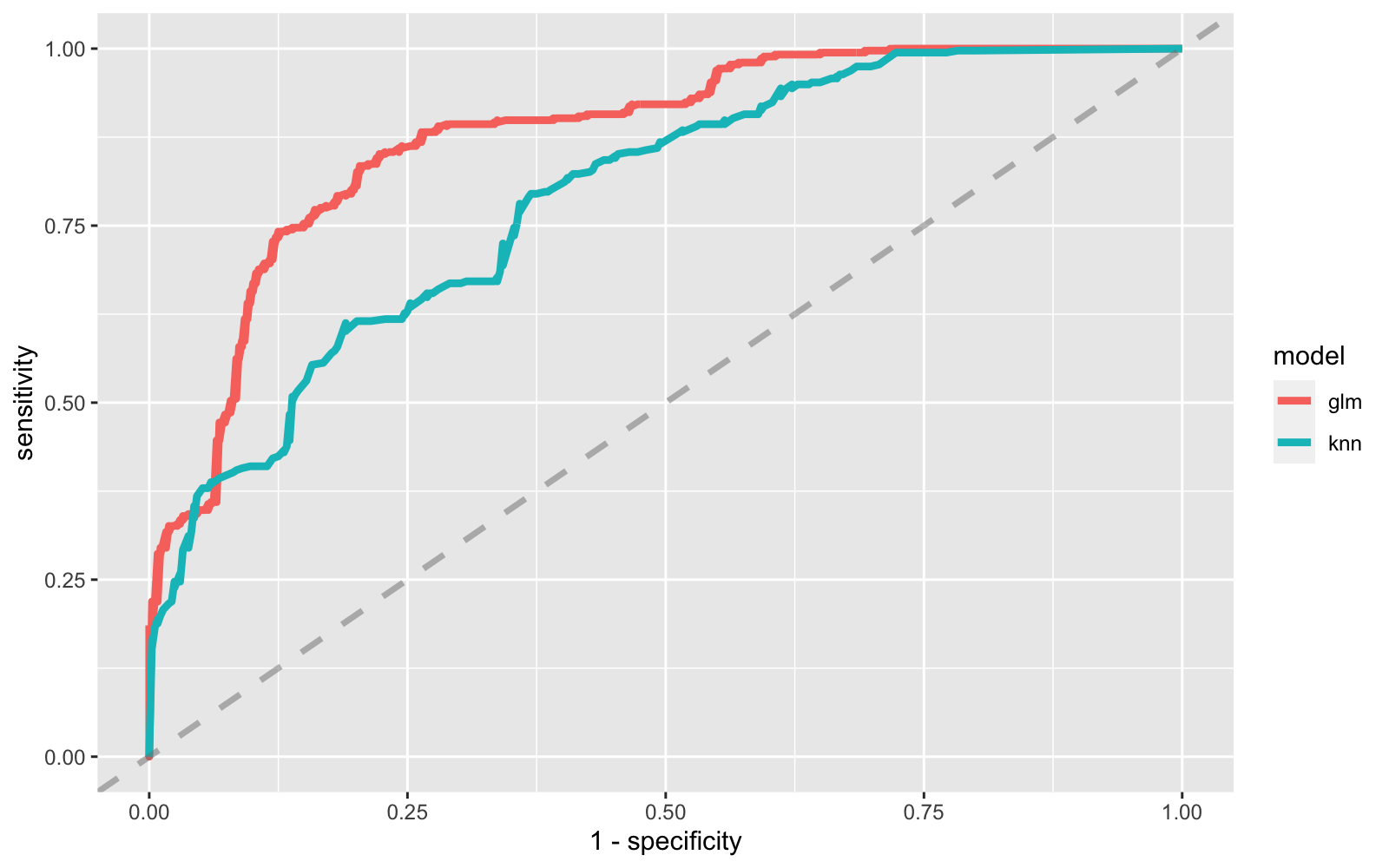Logistic regression model performs a bit better, so we can look at the parameters in detail.

``````glm_fit %>%
tidy() %>%
dplyr::filter(p.value<0.05) %>%
arrange(-estimate)``````
``````## # A tibble: 6 x 5
##   term                           estimate std.error statistic  p.value
##   <chr>                             <dbl>     <dbl>     <dbl>    <dbl>
## 1 writer_John.O.Bryan               0.830     0.198      4.19 2.75e- 5
## 2 writer_Tim.Hedrick                0.679     0.192      3.54 3.97e- 4
## 3 writer_Elizabeth.Welch.Ehasz     -0.750     0.233     -3.21 1.31e- 3
## 4 writer_Michael.Dante.DiMartino   -0.760     0.196     -3.87 1.10e- 4
## 5 book_num                         -1.54      0.295     -5.20 2.00e- 7
## 6 chapter_num                      -2.15      0.260     -8.28 1.24e-16``````

Episodes based on scripts written by John.O.Bryan and Tim Hedrick are more likely to get lower Imdb ratings and episodes based on latest books get higher Imdb ratings .